# Topological Autoencoders

Topology constrained representation learning using autoencoders. Made by Adrish Dey using Weights & Biases

## Introduction

Empowered by the manifold hypothesis, encoding high-dimensional data to low-dimensional latent codes has been extensively used in the machine learning community, both in academia and in industry.
Used everywhere from recommendation systems to data compression, the dimensionality reduction has seen trends ranging from simple linear methods like Principal Component Analysis to complicated Deep Neural Embeddings. And since the beginning of the deep learning revolution, AutoEncoders, with their extremely intuitive architecture, have been at the forefront.
But despite their extensive usage, the latent codes (embeddings) of any dimensionality reduction algorithm can only describe a small neighborhood, induced by the manifold hypothesis, around each encoded vector with little to no information about the global structure of the embedded space. Hence it is generally impossible to describe how the representations are positioned relative to every other vector embedded in that space.
This ICML20 work by Michael Moor et.al. from ETH Zurich, studies the novel problem of dimensionality reduction while preserving the high-dimensional topology of the original data (up to homeomorphism).\\ Paper \longrightarrow \\ Codebase \longrightarrow
Let's get into it:

## Topology of Latent Spaces

In simple terms, topology is a branch of mathematics devoted for studying shapes up to continuous transformations. One intuitive way of understanding what topology refers to, is through the classic topology joke: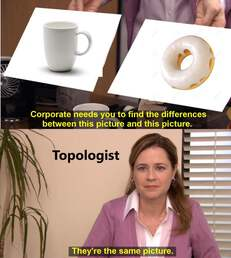Source: Reddit
This rises from the fact that a coffee mug can be continuously deformed to form a donut (torus) and vice-versa. Specifically, we mean that the number of holes in the objects do not change.Source: Wikipedia - Homeomorphism
Formally: two objects are called topologically equivalent if they have equal numbers of d-dimensional holes (holes that can be filled by objects homeomorphic to a d-dimensional sphere), called Betti Numbers denoted by \beta.
Key Takeaway: Betti Numbers serve the purpose of topologically classifying objects. A few common objects, with their Betti Numbers are given below. (\beta_d denotes the d^{th} dimensional betti number.)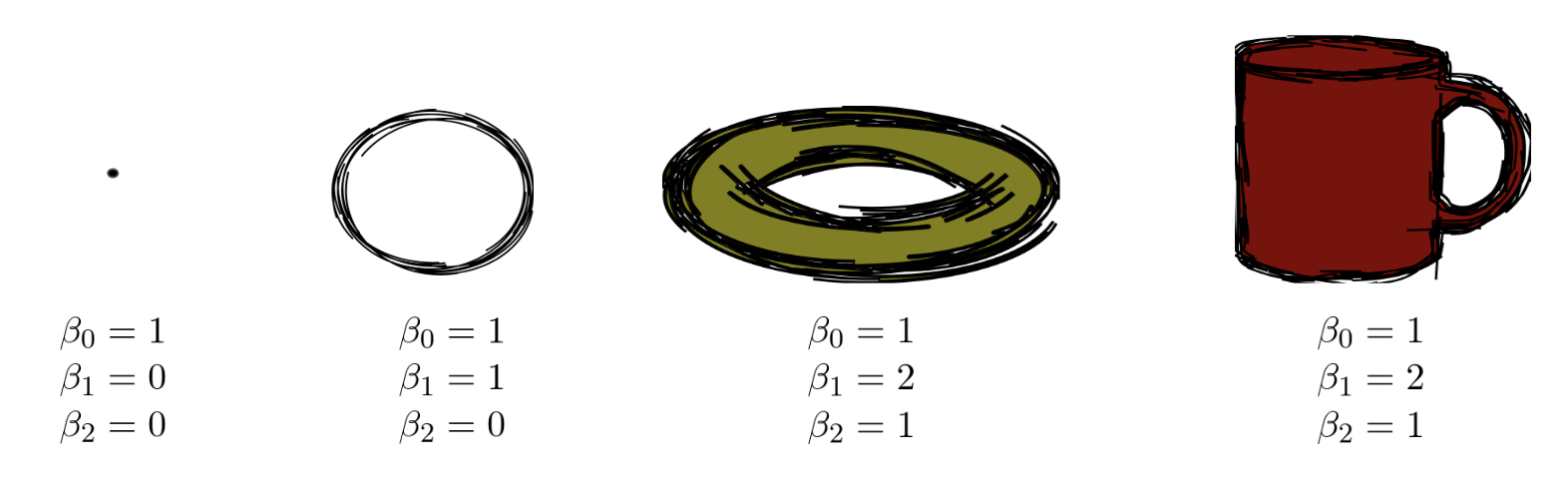Betti Numbers of common objects. (Note: Betti Numbers of torus and mug are the same.)
The primary intuition behind the paper is to enforce a topological regularization on the latent space of AutoEncoders. Hence, it is important to capture the topology of the data manifold from the data samples. Capturing topological descriptors (like Betti Numbers) from point sets is an extremely challenging problem due lack of "connectivity" in high dimensional pointsets. Intuitively, a set of points sampled from some "high-dimensional surface" doesn't encode any information how the surface was originally "connected". Exploring the exponentially large solution space of surfaces (a point can be connected to any other point in the set) is another problem in itself.

### Persistent Homology

Let \mathbf{X} = \left\{ \mathbf{x}_1, \mathbf{x}_2, \mathbf{x}_3, \dots \right\} be a set data points where \mathbf{x}_i \in \mathbb{R}^n. Building upon the union-ball definition of Topological Spaces, a widely used tool from Computational Algebraic Topology called Persistent Homology, approximates a topological descriptor from geometric point sets.
Comprehensively, \epsilon-balls (n-dimensional balls, parameterized by their radius \epsilon) are "grown" around each sample point \mathbf{x}_i, thereby defining a growing neighborhood. Upon intersection of k \epsilon-balls the data points are connected with a k-simplex (k-dimensional triangle). The connectivity formed fills a k-dimensional hole on the original point set.
For futher reference, this growing set of simplicies (formally, simplicial complex) by intersecting growing \epsilon-balls is called a Vietoris-Rips Filtration.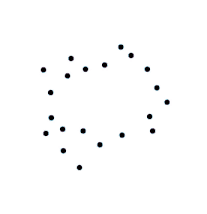Vietoris-Rips Filtration (Source: here)
Finally the birth and death radiuses \epsilon_b and \epsilon_d of each hole is tracked. The primary intuition behind this seemingly complicated algorithm, is the holes that "persists" longer (i.e. high \epsilon_d - \epsilon_b) have higher topological significance. The birth and death radiuses of the holes are plotted onto a chart with values (\epsilon_{b}, \epsilon_{d}), which is called a Persistence Diagram.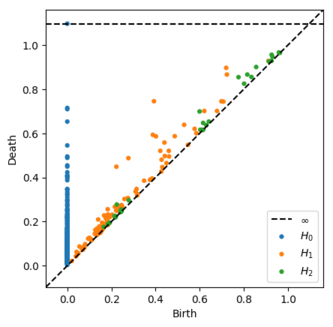Persistence Diagram of the above Filtration. (H_i denotes i-dimensional hole, i.e., i^{th} homology)

### Persistence Diagrams and Topological Loss

One of the primary contributions of this work is the introduction of a novel loss function, capable of comparing topological descriptors (specifically, persistence diagrams) of the data and latent space, in a stable and differentiable manner. In brief, the authors approximate the inherently discrete persistence diagram calculation, using a differentiable relationship on the pairwise distance matrix.
For a Vietoris--Rips Filtration \mathfrak{R}_\epsilon of a dataset \mathbf{X}, the persistent homology PH(\mathfrak{R}_\epsilon), yields the persistence diagrams \{\mathcal{D}_1, \mathcal{D}_2,\dots\} and persistence pairings \{\Pi_1, \Pi_2, \dots\}. The persistence diagrams \mathcal{D}_i is defined by the tuple of birth and death radiuses (\epsilon_b, \epsilon_d) of i^{th} hole. Similarly, the persistence pairings, \Pi_i, is defined by the tuple of "most significant" vertices (vertices of largest simplex which creates / destroyed the hole) for the filtration. Since calculating the persitence diagrams \mathcal{D}_i differentiably, at a scale, is still an active area of research, the authors uses the following expression for approximating the persistence diagrams.
\mathcal{D}_{i} \simeq A_X\left[\Pi_{i}\right]
where A_Xdenotes the pairwise distance matrix of data samples \mathcal{V} or latent space samples \mathcal{Z}.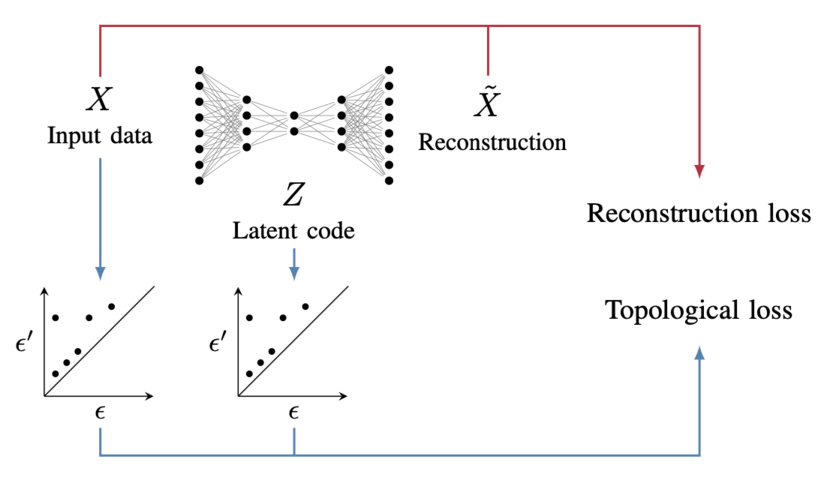Training Setup (extracted from paper)
The final training setup uses this persistence diagram approximation, to define a topological regularization metric (\mathcal{L}_{t}) as shown below.
\mathcal{L}_t := \mathcal{L}_{\mathbf{X}\rightarrow \mathcal{Z}} + \mathcal{L}_{\mathcal{Z}\rightarrow \mathbf{X}}
where \mathcal{L}_{\mathbf{X}\rightarrow\mathcal{Z}} denotes the topological loss term for the encoding procedure, and \mathcal{L}_{\mathcal{Z}\rightarrow\mathbf{X}} denotes the topological loss term form decoding procedure. The mathematical definition of these loss terms are defined as follows.
\mathcal{L}_{\mathbf{X}\rightarrow \mathcal{Z}} := \frac{1}{2} \|A_\mathbf{X}\left[\Pi_\mathcal{Z}\right] - A_\mathcal{Z}\left[\Pi_\mathcal{Z}\right] \|^2
\mathcal{L}_{\mathcal{Z}\rightarrow \mathbf{X}} := \frac{1}{2} \|A_\mathbf{X}\left[\Pi_\mathbf{X}\right] - A_\mathcal{Z}\left[\Pi_\mathbf{X}\right] \|^2
The final loss term is defined as:
\mathcal{L}(X,\,\mathcal{\tilde{X}}) := \mathcal{L}_r(X, \tilde{X}) + \lambda\,\mathcal{L}_t(X, \tilde{X})

## Experiments

The experiments are performed on a topological spheres dataset, as shown below.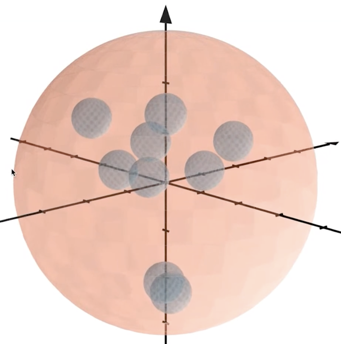Orange hypersphere encapsulates the blue hyperspheres (diagram extracted from ICML Oral Presentation).
The dataset contains a high dimensional hypersphere, which "encapsulates" smaller hyperspheres. As shown below, when the latent space samples are plotted for regular autoencoders (Vanilla AE), it can be observed that every spherical component gets seperated from each other, hence loosing the "encapsulating" structure of the high-dimensional hyperpsheres.
However this is not the case for Topologically Regularized AutoEncoder, where the topological structure of the dataset is preserved in the latent space.

## Conclusion

Enforcing topological regularization to low-dimensional latent space, allows in building richer latent spaces, equivalent to continuous deformations.
This not only contributes towards more reliable visualization of high-dimensional data manifolds, but also opens up various avenues for studying problems involving generative models, implicit representations, geodesic interpolation, etc. The novelty of this paper also extends in being one of the very few attempts to bridge Computational Algebraic Topology, Topological Data Analysis with main-stream Deep Learning.
With that said, this rise in interest for Topology and Geometry in Deep Learning, would surely give a fresh perspective to various unexplained problems and wonders Deep Learning offers.